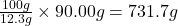## A chemistry student needs 90.00 g of thiophene for an experiment. She has available 0.50 kg of a 12.3% w/w solution of thiophene in ethanol.

Question

A chemistry student needs 90.00 g of thiophene for an experiment. She has available 0.50 kg of a 12.3% w/w solution of thiophene in ethanol.
Calculate the mass of solution the student should use. If there’s not enough solution, press the ‘No solution” button
Round your answer to 3 significant digits. Put the answer in terms of the amount of grams.

in progress 0
2 months 2021-07-30T10:30:25+00:00 1 Answers 7 views 0

Explanation:

We are given:

Given mass of thiophene for the experiment = 90.00 g

12.3% w/w solution of thiophene

This means that 12.3 g of thiophene is present in 100 g of solution

Applying unitary method:

If 12.3 g of thiophene is present in 100 g of solution

So, 90.00 g of thiophene will be present inof solution

Converting it into kilograms:

1 kg = 1000 g

So, 731.7 g = 0.7317 kg

As the given amount of solution is 0.50 kg which is less than the required amount.

Thus, there is not enough solution the student should use.